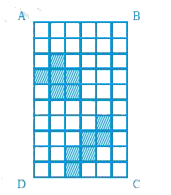# In Fig. 6.18 each square is of unit length (a) What is the perimeter of the rectangle ABCD? (b) What is the area of the rectangle ABCD? (c) Divide this rectangle into ten parts of equal area by shading squares. (Two parts of equal area are shown here) (d) Find the perimeter of each part which you have divided. Are they all equal?Updated on:21/07/2023